We have been busy at MathPlus school, working hard with our students and finding innovative, fun and educational classes to add to our offerings. Please see below for a sampling of what our students are working on.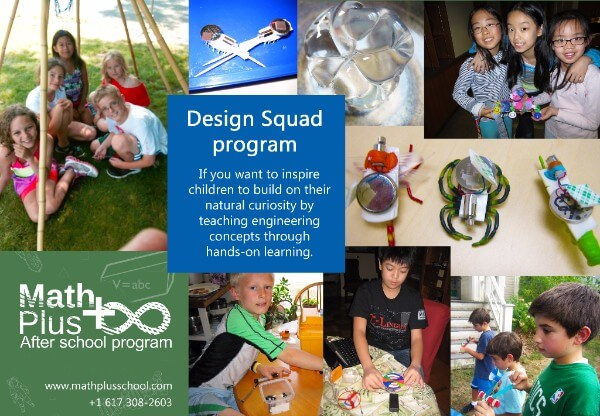Every class has been an exciting one with Mrs. Nic. Students have learned about electrical engineering by making small “BrushBots” that can move and spin. They have studied how physical properties are affected by changing temperatures by making their own “Natural Neosporin.” Most recently, the student studied bio-inspiration to understand how animals’ bodies and behaviors make our lives better by focusing on the cone snail shell and Gecko Tape (“Rip Wrap”). To further this lesson, the class was introduced to two lizards so they can observe the head, rear feet and tail. Space is still available for this class, please contact us to have your student join in on the fun!

## Preschool Math Class

The Preschool Math class is growing and learning every day. The focus so far has been on:

• Number sense (e.g., the numeral “4” represents four objects, which is greater than 3 and less than 5: How many more, how many less, how to make them equal)
• Working with a number line, count by 2, count by 10
• Geometry (e.g., patterns and shapes, each with unique features), continue Geometry patterns
• Making classifications of objects by color, shapes, size
• Describing pictures using “above”, under”, “left” ,”right”, above”
• Forming the definition for “right “and left”.
• Building pairs and counting objects by pairs.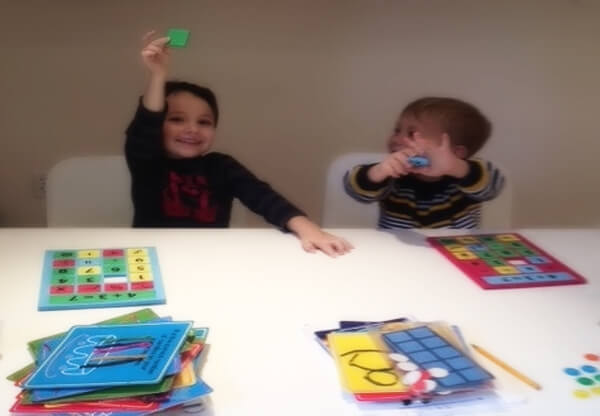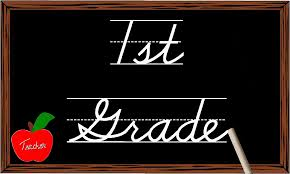Students in the 1st grade math class have been focusing on:

• Measurements: sm., inch, lb.
• Logic: to the right, to the left. Word logic problems
• Solving basic operations using a number line
• 3D Shapes: learning the definitions
• Simplifying expressions with positive and negative numbers
• Using the signs for inequality
• Showing all the different ways to make the following numbers:1-10
• Classification of geometry shapes by color, shape and size
• Finishing patterns

Students in 2nd grade math class have been focusi

• Basic multiplication
• Addition and subtraction of 2 digit and 3 digit numbers
• Word problems and math modeling
• Logic: pairs and patterns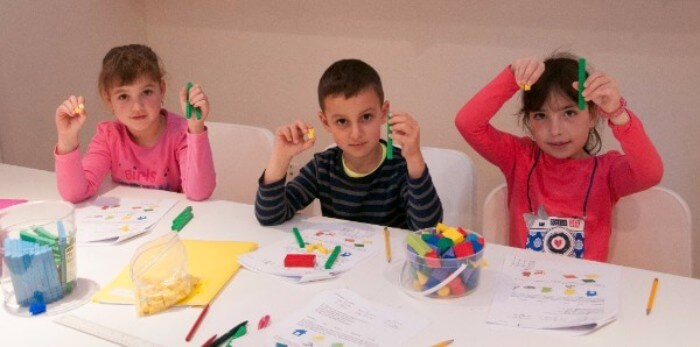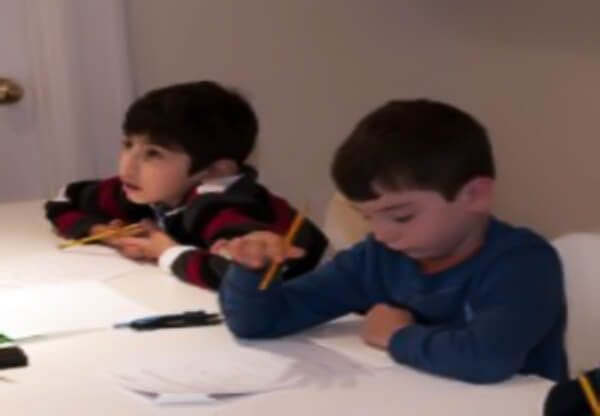Students in 3rd grade math class have been focusing on:

• Defining sets, elements and subsets
• Logic word problems
• Geometry: lines (parallel, perpendicular and intersecting), point, line segment, ray, faces (curved, flat or flat and curved), triangles and planes
• 3D shapes

Students in 4th grade math class have been focusing on:

• Order of operations
• Prime numbers
• Negative numbers
• Fractions
• Divisibility tests
• Long division and multiplication
• Cartesian coordinate system
• Solving equations using auxiliary drawings
• Making a Venn diagram for sets
• Algebra
• Expressions and equations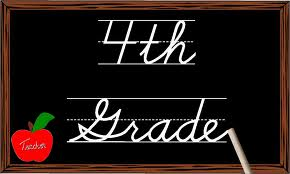Students in 6th grade math class have been focusing on:

• Theory of sets on the example of natural, rational and real numbers
• Logical operations: and, or, xor, implies
• Geometry: triangle constructions with a ruler and compass